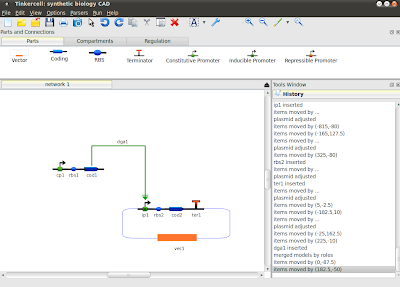## Monday, November 22, 2010

### Using the plot tool from pluginsIn addition to normal line plots, the plot tool features scatter plots, surface plots, and histograms. It can also to multiple plots. All of these features can be accessed from C++, C, Python, or Octave. Here is some code:

In C:
tc_matrix m = tc_plotData(i); //get data in ith plot window

tc_multiplot(2,1); //plot 2 graphs
tc_plot(m, "title"); //plot normal plot
tc_scatterplot(m, "title"); //scatterplot
tc_hist(m, "title"); //histogram
tc_surfacePlot(m, "title"); //3D plot - m must have 3

In Python:

m = tc_plotData(i); //get data in ith plot window
m2 = fromTC(m); //convert to python array

m = toTC(m2); //convert to tinkercell matrix
tc_multiplot(2,1); //plot 2 graphs
tc_plot(m, "title"); //plot normal plot
tc_scatterplot(m, "title"); //scatterplot
tc_hist(m, "title"); //histogram
tc_surfacePlot(m, "title"); //3D plot - m must have 3

In Octave:

m = tc_plotData(i); //get data in ith plot window
m2 = fromTC(m); //convert to octave matrix

//currently there is no way to convert to tinkercell matrix
//you will have to use tc_setMatrixValue to set each i,j-th
//value, i.e. write a double for-loop
tc_multiplot(2,1); //plot 2 graphs
tc_plot(m, "title"); //plot normal plot
tc_scatterplot(m, "title"); //scatterplot
tc_hist(m, "title"); //histogram
tc_surfacePlot(m, "title"); //3D plot - m must have 3

C++ (more code and more flexibility)
//get the plot tool
PlotTool * plot = static_cast (mainWindow->tool("plot"));

//get list of all plot windows inside the plot tool
QList<> plotWidgets = plot->plotWidgets();

//get the data from the i-th plot window
NumericalDataTable data = plotWidgets->data();

//plot multiple graphs or plot on top of existing plot
plot->hold(true);
plot->overplot(true);

//plot data
plot->plot(data, "title");

//plot specific type of graph
int xaxis = 0; //index to use for x-axis

//specify plot type: Plot2D, SurfacePlot, HistogramPlot, ScatterPlot, BarPlot, Text

plot->data(data, "title", xaxis, PlotTool::BarPlot);

## Friday, November 12, 2010

### TinkerCell "lite"

MainWindow now has a new static field called PROGRAM_MODE. This is an optional field used to indicate the "mode" of the program. Different plug-ins can choose to behave differently based on the program mode. For example, setting the mode to "lite" makes the CatalogTool load minimal tabs at the top and prevents some other tools from showing themselves.